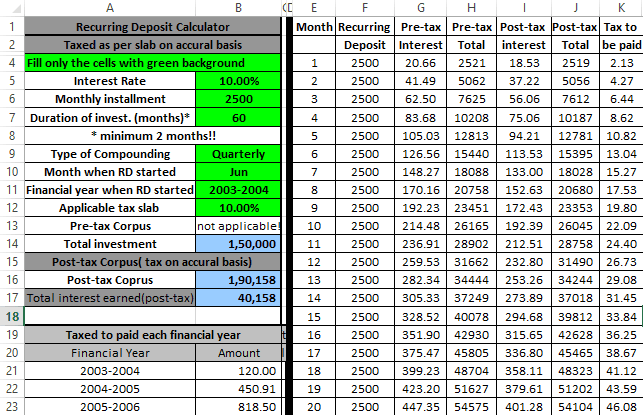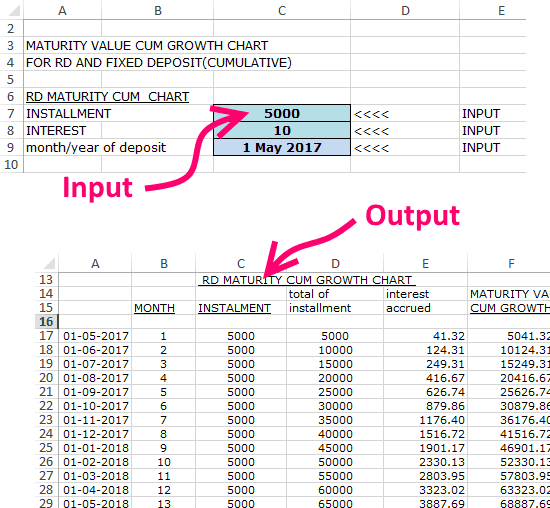Editor Ratings:
User Ratings:
[Total: 2 Average: 4.5]

Here are 3 Free Recurring Deposit Calculator software that let you calculate interest earned, and final maturity amount for recurring deposit. These RD calculators are very easy to use, and give you a simple view where you can easily see the maturity amount that you will get if you start a RD with specific monthly deposits and interest rate. Some of these also show monthly break-up for recurring deposits.

One of these is a simple software, that just shows the final maturity amount, without showing the monthly growth of recurring deposit. Other two are Excel based Recurring deposit calculators that show complete monthly break-up.

In case you don’t know, Recurring Deposits are a special type of Fixed Deposits or Term Deposits that are primarily popular in India. In this, instead of depositing a lump sum account, user deposits a fixed amount every month, and gets the same rate of interest as a Fixed Deposit.

## Interest CalculatorInterest Calculator (Homepage) is a very basic free Recurring Deposit calculator. It is truly as simple as it gets. You just need to enter monthly investment amount, Yearly interest rate, and number of years for which you will hold the recurring deposit. It will then give you the maturity value of your recurring deposit.

This free RD calculator lets you choose the interest calculation period. You can choose between monthly interest payout, quarterly interest, or halfyearly interest.

Once you have entered all the details, just click on “Calculate” button, and it will show the maturity amount. That’s all it shows. I really wish it showed monthly break-up as well.

You can download this software as a rar file from the link above. Just extract the rar file (I used 7-Zip for that). In the extracted file, there is an exe file. Just double click on it to install it. Once installed, you will see the interface like above. Enter all the values to get the result.

Apart from using it as Recurring Deposit calculator, you can also use it to calculate Compound Interest, and EMI calculation (though you are better off using a Mortgage calculator for that).

## Excel RD CalculatorOneMint.com has published a nice Excel RD calculator that is very feature rich, and shows a lot of details. You can enter RD Interest Rate, Monthly Installment, and Duration, and then it will show the Maturity Amount. You can also choose compounding period between monthly, quarterly, half yearly, and yearly. However, in addition to that, it actually also shows monthly break-up of the interest earned so that you can see how your investment in recurring deposit is growing monthly.

This Excel Recurring Deposit Calculator does not stop just there. It also lets you calculate Tax on your Recurring Deposit. You can choose the tax slab that you fall in, and it will then compute tax for you, and will show how much monthly tax has to be paid as well as overall tax deduction. In that case, it also shows the final maturity amount after tax.

I really like this Excel based RD calculator for the comprehensive information it provides. The month wise break-up is especially exciting for me, as I can see how my RD corpus grows month over month with time.

## Another Excel Based RD Calculator:I found another Excel based Recurring Deposit Calculator that shows monthly break-up of Recurring Deposit interest accumulated. However, it is not as feature rich as the one I mentioned above, but it gets the task done. You can get it from here (it will directly start the download). In this excel, you need to provide monthly installment amount, interest rate, and start month / year. It will then show the recurring deposit value for next 10 years. It will show month-wise break-up for this duration. For each month, you can see total amount deposited by you till date, total interest accrued as of that month, and maturity value (in case the Recurring deposit will mature in that month). There is no option to specify RD period, or compounding method (monthly / quarterly etc.).

To use this RD calculation excel, go to its “Input” tab and provide the values in the cells that are highlighted in Blue. The Input tab has section for FD as well, you can ignore that.

After that, go to “Maturity Cum Growth Chart” tab, and you can see the month-wise breakup of your recurring deposit.

I don’t really like this excel calculator a lot, especially because the previous Excel calculator is simpler, provides more options, and data is better laid out.

### Closing Words:

These Recurring Deposit calculators make it extremely easy to calculate maturity value of Recurring Deposit, as well as monthly growth. My personal favorite in this list is the Excel based RD calculator that I listed at number 2 above, because it has everything I would expect from a Excel based Recurring Deposit calculator.

 Editor Ratings: User Ratings:[Total: 2 Average: 4.5]